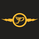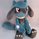# Risk Position Sizing tool using Coefficient of Variation525 views
A way to manage portfolio risk using relative standard deviation, also known as coefficient of variation. This tool tells you how much of each stock in shares and in value to buy adjusted for their volatility risk for a given starting account capital. A problem many people have is how to diversify an account and adjusting it for the risk involved in each equity. Many would put in an equal amount of capital value into each share but is it really equal if some equities have more risk than others? A solution is to adjust the portfolio by giving less weight to those that are more volatile or risky. It's done by using a starting percent of the account, preferably a small percent of it, and buying up shares with that same amount for each equity. Each equity will also be divided by the COV to risk adjust the portfolio by giving less weight to the more volatile stocks. This is done until as much of the initial capital in the account as possible is spent.

COV is how far away the price is from the mean or average. The further the price is from the mean the more risk or volatility there is. It uses standard deviation in its calculation. The problem with SD and ATR is that they are not relative to the past or to other equities to compare to. An application where COV can be used is risk portfolio management formulas. This does not take into account correlation or other equation parts in some portfolio management formulas but only the risk or volatility , the default volatility length is mostly arbitrary, and the lower risk stocks may end up being the slowest in performance.

The text label will show how many shares will be bought and how much value each equity will have. At the end it will show the initial capital that was started off with, the total shares bought, the total value of all the shares, and the amount of capital left over. If the sources are not blank then they will be used, to blank them you will need to reset the settings to default otherwise they might still be read. If you want to add more than the given 10 equity spaces to the portfolio then you will need to add in the code manually and add it to the chart. The denominator is perhaps the important part in these types of risk position sizing tools, you can change to other things such as risk-reward ratio instead of volatility or change the volatility type, etc.
Release Notes: fixed a problem with the volatility somehow existing despite empty input symbol sources which ended up throwing things off
Open-source script

In true TradingView spirit, the author of this script has published it open-source, so traders can understand and verify it. Cheers to the author! You may use it for free, but reuse of this code in a publication is governed by House Rules. You can favorite it to use it on a chart.

Want to use this script on a chart?Hi there, is me again... sorry to bother you but noticed when I do 1,2 or 3..etc symbols calculation is different than doing all 10 symbols which is more accurate based on deviation
You have to use all 10 symbols so that calculations based on deviation to work otherwise it uses all capital and the other settings like lenght or risk become unusablePiticu
@Piticu, hi thanks for the comments. The goal is to spend all the money and let this math tool tell you how to distribute all of it based on the volatility of each stock. It's suppose to spend all the money no matter how many inputs you give it or as much as possible depending on what starting risk percent you gave it. The first and second pictures show that the more volatile ones like bitcoin received less capital bought into them than the others because it's likely one of the more riskier assets in a portfolio(as many people would agree). As long as the number and value of each share adds up to the bottom totals, then it's likely working fine.Hi mate
First of all thank you for posting this indicator and many others, all very helpful and simple done, this one looks is more complicated but is even more usefully.
I found an issue that may be a simple fix, idk...
Same setting , same timeframe on a 2 window chart to compare but different symbols is giving different results